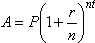# Compound Interest : Java Program In 5 Simple Ways | Programs

How to write a Java program to calculate compound interest? Here we share the code in five different formats to find compound interest program. Using standard values, using recursion, using command line arguments, using while loop, for loop, and using function method etc.

We can even write the following Java code to find compound interest in more than 10+ ways as a beginner we share only the basic versions here. If you have any doubts related to the following code, then do leave a comment here. Here is the complete list of beginners programs and java interview programs. Also, check out the core java interview questions.

• How to calculate compound interest in math?

In simple, compound interest is a interest on interest. It’s the result of reinvesting.

• What’s the formula to find the compound interest?Where,

• A = ” Ending Amount “
• P = ” Principal “
• R =” Interest Rate “
• N = ” Number of compounding a year “
• T = ” Total Number Of Years “

Here we go Basic java program with standard values ( Formula-based ) to print compound Interest followed by recursion and control statements. At the end of the codes, we embedded an online execution tool where you can execute and print the output for the following programs. Check out the sample simple CI program -1 below with outputs as well.

Find CI using standard values

• ( Using standard values ) by using the simple/basic math formula you can write any program based on the following example. #Formula based Program#. Also, check the output and execute the following program at the end of the post.
Output ( Standard Values ) :

Using Command Line Arguments

Output ( Using command line arguments ) :

Using  For Loop

Here we go another basic and simple method to print CI values, java program to calculate compound interest using For loop.

Executing a set of statements repeatedly is known as looping. We have written an article about for loop here. Check out. For loop is the most used loop in Java, where it can work out with both while and do while. Check out the program below with outputs.

Output ( Using For Loop ) :

Using While Loop

• Another simple program to print CI values, here we used a while loop syntax to find the compound interest using while loop.
• The difference between for loop and while loop is nothing, but as per universal laws, the execution of statement differs with that code thereby all looping will have a different syntax which can impact on the execution of the statement.
• The definition of while loop is executing a set of statements repetitively until the condition is true. Check out the program.
Output ( Using While Loop ) :

Using Recursion

• Another method, using recursion. So basically what is recursion, recursion is a method calls itself repeatedly or a method in java that calls itself is called a recursive method. Check out the following program using recursion.
Output ( Using Recursion ) :

We are working on to embed the online execution tool. The online execution tool will help you to learn and practice more programs. You can even execute the above codes and find out how it works in each different case using static, for loop, while loop, and using recursion.

Here is the quick reference links: 100+ Beginners programs, Java interview programs and interview question and answers.

More Tutorials :

If you want any custom program, do contact us our expert developers will ready to handle any project or source codes for your project or assignment.

x

## X Star Pattern Java Program – Patterns

Java program to print X star pattern program – We have written the below print/draw ...